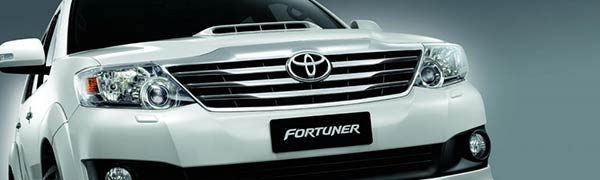Fortuner Fortunes

Calculus Level 3Fortuner, the latest SUV by Toyota Motors, consumes diesel at the rate of $\frac{1}{400} \times [ \frac{1000} { X} +X]$ litres per km, when driven at the speed of $X$ km per hour. If the cost of diesel is Rs 35 per litre and the driver is paid at the rate of Rs 125 per hour, what is the approximate optimal speed (in km per hour, as an integer) of Fortuner that will minimize the total cost of a round trip of 800 kilometers?

×